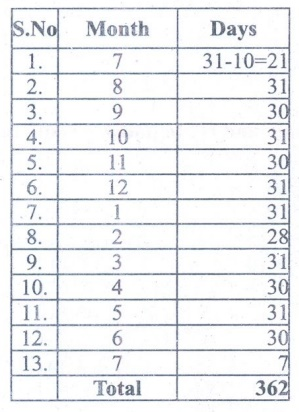Home | | Maths 4th Std | Exercise 4.1 (time, date, calendar)

# Exercise 4.1 (time, date, calendar)

Text Book Back Exercises Questions with Answers, Solution : 4th Maths : Term 3 Unit 4 : Time : Exercise 4.1 (time, date, calendar)

Exercise 4.1

1. Find out the days between manufacturing and expiry date of a medicine given below.Mfg Date = 09.07.2013

Exp. Date = 08.08.20152. Select a month in the calendar and find out any creative pattern of your own in the numbers.

6 13 20

7 14 21

8 15 22

6 + 14 + 22 = 42

8 + 14 + 20 = 42

13+14+15 = 42

17+14+21 = 42

Tags : Time | Term 3 Chapter 4 | 4th Maths , 4th Maths : Term 3 Unit 4 : Time
Study Material, Lecturing Notes, Assignment, Reference, Wiki description explanation, brief detail
4th Maths : Term 3 Unit 4 : Time : Exercise 4.1 (time, date, calendar) | Time | Term 3 Chapter 4 | 4th Maths